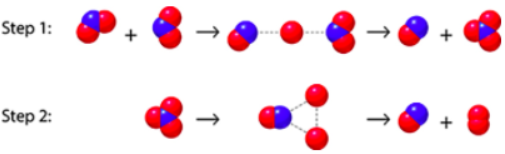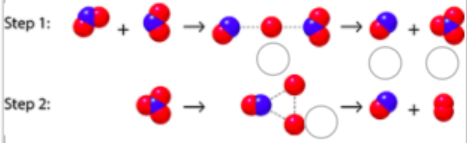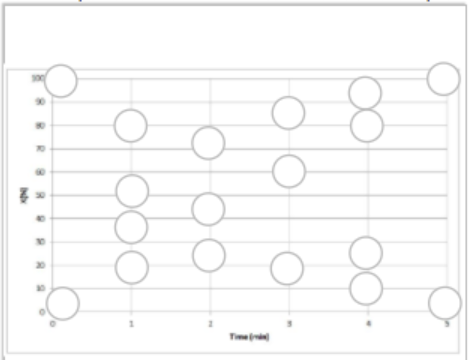# Problem: Given this particulate model, answer the following questions. The blue atoms are nitrogen, and the red atoms are oxygen.Label the intermediate(s) and transition state(s) in the reaction mechanism.A. First intermediate formedB. Second intermediate formedC. First transition state formedD. Second transition state formedE. Third transition state formedF. ProductWith regard to molecularity, the first step in the reaction is ______a) termolecular b) bimolecularc) none of thesed) unimolecularConsider the equation Y+B → X where the formation of X follows second-order kinetics. The reactant is completely consumed in 5 minutes.Plot six data points to show how the concentration of the product X changes over time for the reaction shown below.A. t = 0 minB. t = 1 minC. t = 2 minD. t = 3 minE. t = 4 minF. t = 5 min

###### FREE Expert Solution

Label the intermediate(s) and transition state(s) in the reaction mechanism

reaction intermediate – molecular entity that is formed from the reactants and reacts further to give the directly observed products of a chemical reaction

transition state – configuration along the reaction coordinate

82% (154 ratings)###### Problem Details

Given this particulate model, answer the following questions. The blue atoms are nitrogen, and the red atoms are oxygen.Label the intermediate(s) and transition state(s) in the reaction mechanism.A. First intermediate formed

B. Second intermediate formed

C. First transition state formed

D. Second transition state formed

E. Third transition state formed

F. Product

With regard to molecularity, the first step in the reaction is ______

a) termolecular

b) bimolecular

c) none of these

d) unimolecular

Consider the equation Y+B  X where the formation of X follows second-order kinetics. The reactant is completely consumed in 5 minutes.

Plot six data points to show how the concentration of the product X changes over time for the reaction shown below.A. t = 0 min

B. t = 1 min

C. t = 2 min

D. t = 3 min

E. t = 4 min

F. t = 5 min